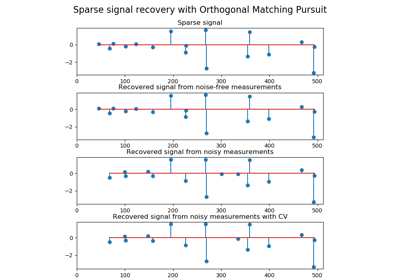# sklearn.linear_model.OrthogonalMatchingPursuit¶

class sklearn.linear_model.OrthogonalMatchingPursuit(n_nonzero_coefs=None, tol=None, fit_intercept=True, normalize=True, precompute='auto')[source]

Orthogonal Matching Pursuit model (OMP)

Read more in the User Guide.

Parameters
n_nonzero_coefsint, optional

Desired number of non-zero entries in the solution. If None (by default) this value is set to 10% of n_features.

tolfloat, optional

Maximum norm of the residual. If not None, overrides n_nonzero_coefs.

fit_interceptboolean, optional

whether to calculate the intercept for this model. If set to false, no intercept will be used in calculations (i.e. data is expected to be centered).

normalizeboolean, optional, default True

This parameter is ignored when fit_intercept is set to False. If True, the regressors X will be normalized before regression by subtracting the mean and dividing by the l2-norm. If you wish to standardize, please use sklearn.preprocessing.StandardScaler before calling fit on an estimator with normalize=False.

precompute{True, False, ‘auto’}, default ‘auto’

Whether to use a precomputed Gram and Xy matrix to speed up calculations. Improves performance when n_targets or n_samples is very large. Note that if you already have such matrices, you can pass them directly to the fit method.

Attributes
coef_array, shape (n_features,) or (n_targets, n_features)

parameter vector (w in the formula)

intercept_float or array, shape (n_targets,)

independent term in decision function.

n_iter_int or array-like

Number of active features across every target.

Notes

Orthogonal matching pursuit was introduced in G. Mallat, Z. Zhang, Matching pursuits with time-frequency dictionaries, IEEE Transactions on Signal Processing, Vol. 41, No. 12. (December 1993), pp. 3397-3415. (http://blanche.polytechnique.fr/~mallat/papiers/MallatPursuit93.pdf)

This implementation is based on Rubinstein, R., Zibulevsky, M. and Elad, M., Efficient Implementation of the K-SVD Algorithm using Batch Orthogonal Matching Pursuit Technical Report - CS Technion, April 2008. https://www.cs.technion.ac.il/~ronrubin/Publications/KSVD-OMP-v2.pdf

Examples

>>> from sklearn.linear_model import OrthogonalMatchingPursuit
>>> from sklearn.datasets import make_regression
>>> X, y = make_regression(noise=4, random_state=0)
>>> reg = OrthogonalMatchingPursuit().fit(X, y)
>>> reg.score(X, y)
0.9991...
>>> reg.predict(X[:1,])
array([-78.3854...])


Methods

 fit(self, X, y) Fit the model using X, y as training data. get_params(self[, deep]) Get parameters for this estimator. predict(self, X) Predict using the linear model. score(self, X, y[, sample_weight]) Return the coefficient of determination R^2 of the prediction. set_params(self, \*\*params) Set the parameters of this estimator.
__init__(self, n_nonzero_coefs=None, tol=None, fit_intercept=True, normalize=True, precompute='auto')[source]

Initialize self. See help(type(self)) for accurate signature.

fit(self, X, y)[source]

Fit the model using X, y as training data.

Parameters
Xarray-like, shape (n_samples, n_features)

Training data.

yarray-like, shape (n_samples,) or (n_samples, n_targets)

Target values. Will be cast to X’s dtype if necessary

Returns
selfobject

returns an instance of self.

get_params(self, deep=True)[source]

Get parameters for this estimator.

Parameters
deepbool, default=True

If True, will return the parameters for this estimator and contained subobjects that are estimators.

Returns
paramsmapping of string to any

Parameter names mapped to their values.

predict(self, X)[source]

Predict using the linear model.

Parameters
Xarray_like or sparse matrix, shape (n_samples, n_features)

Samples.

Returns
Carray, shape (n_samples,)

Returns predicted values.

score(self, X, y, sample_weight=None)[source]

Return the coefficient of determination R^2 of the prediction.

The coefficient R^2 is defined as (1 - u/v), where u is the residual sum of squares ((y_true - y_pred) ** 2).sum() and v is the total sum of squares ((y_true - y_true.mean()) ** 2).sum(). The best possible score is 1.0 and it can be negative (because the model can be arbitrarily worse). A constant model that always predicts the expected value of y, disregarding the input features, would get a R^2 score of 0.0.

Parameters
Xarray-like of shape (n_samples, n_features)

Test samples. For some estimators this may be a precomputed kernel matrix or a list of generic objects instead, shape = (n_samples, n_samples_fitted), where n_samples_fitted is the number of samples used in the fitting for the estimator.

yarray-like of shape (n_samples,) or (n_samples, n_outputs)

True values for X.

sample_weightarray-like of shape (n_samples,), default=None

Sample weights.

Returns
scorefloat

R^2 of self.predict(X) wrt. y.

Notes

The R2 score used when calling score on a regressor will use multioutput='uniform_average' from version 0.23 to keep consistent with r2_score. This will influence the score method of all the multioutput regressors (except for MultiOutputRegressor). To specify the default value manually and avoid the warning, please either call r2_score directly or make a custom scorer with make_scorer (the built-in scorer 'r2' uses multioutput='uniform_average').

set_params(self, **params)[source]

Set the parameters of this estimator.

The method works on simple estimators as well as on nested objects (such as pipelines). The latter have parameters of the form <component>__<parameter> so that it’s possible to update each component of a nested object.

Parameters
**paramsdict

Estimator parameters.

Returns
selfobject

Estimator instance.

## Examples using sklearn.linear_model.OrthogonalMatchingPursuit¶